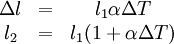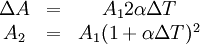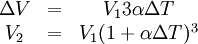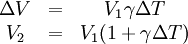# What is a longitudinal extension

Under Thermal expansion one understands the change in the geometric dimensions (length, area, volume) of a body, caused by a change in its temperature. The reverse of this process by cooling is often called Heat shrinkage designated.

### Impact and Applications

Thermal expansion is always accompanied by a change in density. In the case of fluid bodies, this can lead to changed pressure conditions. In particular, the resulting convection manifests itself in ocean currents and air currents and is part of the weather. This is used in thermal power plants and in gliding. Other examples of thermal expansion uses include bimetal strips, many types of thermometers and thermostats, the Stirling engine, all internal combustion engines, and hot air balloons.

If there are different thermal expansions in a body or in mechanically connected bodies, mechanical stresses can arise, which in extreme cases can lead to damage or destruction of a component. This is impressively demonstrated in the so-called bolt explosion test. Certain dimensions change in the opposite direction to the change in length of the components. Thus, constructively provided distances between components can be reduced or closed as they expand. A difference in expansion can be caused by a temperature difference or a combination of materials with different thermal expansion behavior.

Architects, civil engineers and designers keep different thermal expansions low by using suitable materials. In addition or as an alternative, expansion joints, sufficient play between components, compensation of the differences in size using expansion joints are used. Position deviations caused by thermal expansion in electronically controlled machines, such as robots, can also be compensated for by control technology.

### Heat shrinkage

The term heat shrinkage is used for various processes.

• Because during casting, the warm material shrinks after it has solidified in the mold, giving off heat, this is sometimes referred to as heat shrinkage. This is due to thermal expansion. The measure for heat shrinkage is the shrinkage, which is specified as a percentage of the finished size depending on the material or based on absolute dimensions in the respective length unit. The degree of shrinkage is largely determined by the material, since the second influencing variable, the temperature difference, is largely determined by the material via the appropriate casting temperature.
• On the other hand, however, heat shrinkage is often also referred to as deformation due to heating, which is desirable with shrink tubing and, as with other permanent material shrinkage due to the action of heat, is not caused by thermal expansion.

### Causes of thermal expansion

In a solid, every single atom oscillates around a point of equilibrium. If it were harmonic oscillations, the distance between the atoms would have to remain the same on average as the equilibrium distance, because the atoms oscillate to the same extent in the direction of a neighboring atom and in the opposite direction. Therefore, the thermal expansion cannot be described with the approximation of the harmonic potential, but it must be taken into account that the potential energy increases more when two atoms approach each other than when they move away from each other. Due to the steeper potential curve, the deflection in the direction of a closer neighboring atom is smaller and at the same time the restoring force is greater than in the oscillation away from the neighboring atom (or in the direction of an atom further away); as a result, the atom spends less time in the vicinity of the neighboring atom, the distances between the atoms are on average larger than the equilibrium distance. If the vibrations take place with low energies, the potential is still relatively symmetrical; the higher the energies, the further the atoms vibrate in the asymmetrical area of ​​the potential. Higher energies are present at higher temperatures, therefore expansion occurs when heated.

In the case of gases, the pressure increases at constant volume with increasing temperature, because the higher particle energy means more momentum per particle z. B. is delivered to a vessel wall, and the speed of the particles is higher, which leads to more impacting particles per unit of time. If the pressure is to remain constant, the volume must be increased so that the lower particle density compensates for the effects mentioned above. In the case of gases whose behavior deviates from that of the ideal gas, forces of attraction between the gas particles, which reduce thermal expansion, and the volume of an individual particle also play a role.

In liquids, thermal expansion has the same causes as in gases, except that it is greatly reduced by the forces of attraction between the particles.

### Coefficient of thermal expansion

The coefficient of thermal expansion is a parameter that describes the behavior of a substance in a certain temperature range. A distinction is made between the coefficient of linear expansion α (also linear thermal expansion coefficient) and the room expansion coefficient γ (spatial or Volume expansion coefficient).

### Equations

Since the thermal expansion, especially in the case of solid bodies, is strongly dependent on the lattice structure or the bond conditions, the linear equations only represent approximations in the range of the standard conditions. For example, sudden changes can occur at transitions in the crystal structure or non-linearities become apparent in the case of larger temperature differences, so that equations of the second or even higher order with two or more coefficients must be used.

### Solid

length surface volume### liquids### Gases

Gases also tend to expand when the temperature rises. However, a cubic expansion coefficient corresponding to the γ of the formulas for liquids can only be defined for a certain starting temperature. For an ideal gas at an outlet temperature of 0 ° C it is

γ = 1 / 273.15K-1

In general, according to the equation of state for ideal gases under constant pressure, V ~ T, i.e. H. V2 = V1 * T2 / T1. This means that when the absolute temperature is doubled, the volume also doubles.

### Formula symbol

 A. surface l length ΔT Temperature difference in K V. volume α Coefficient of linear expansion in K-1 γ Room expansion coefficient in K-1

### particularities

Some materials such as zirconium tungstate or carbon fiber reinforced plastics (CFRP) can have a negative coefficient of expansion. In the case of CFRP, this is anisotropic (direction-dependent). In some temperature ranges, water has a negative coefficient of expansion (water anomaly).

Category: Materials Science